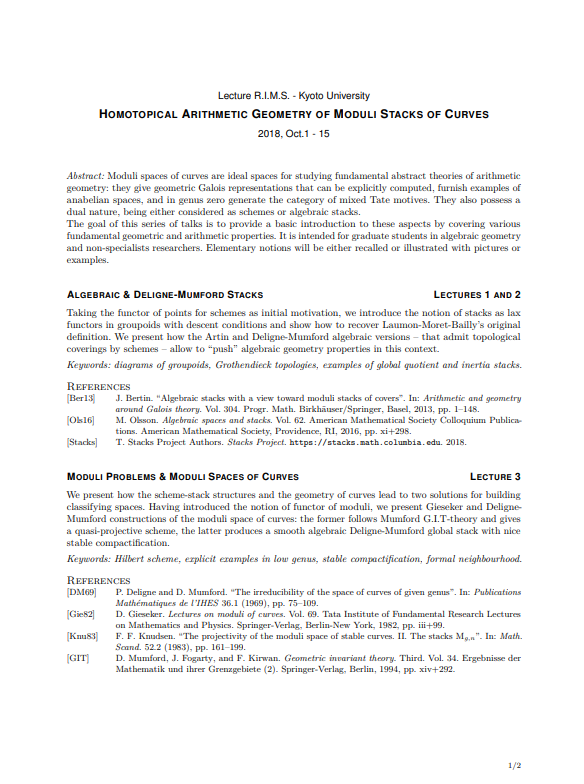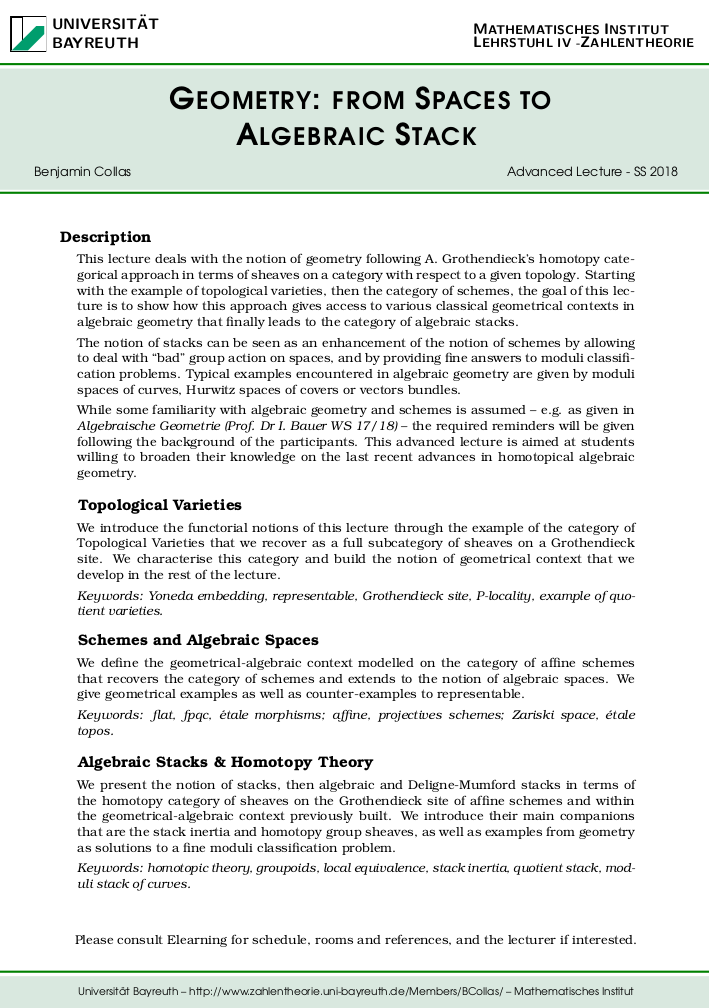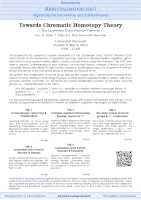### Arithmetic Homotopy of Moduli Stack of Curves, RIMS Kyoto, 2018.

Moduli stacks of curves are ideal spaces in the study of geometric Galois representations and motivic theory. In these series of lectures, we present fundamental geometric and arithmetic properties of these spaces -- I & II - Algebraic & Deligne-Mumford Stacks, III - Moduli Problems & Moduli Spaces of Curves, IV - Fundamental Group & Arithmetic, V - Motivic Theory for Moduli Stack of Curves -- the goal being to introduce some elements of arithmetic homotopy theory (etale homotopy type, homotopical stacks, Morel-Voevodsky motivic homotopy).

Keywords: Diagrams of groupoids, algebraic stacks; GIT-theory, Gieseker & Deligne-Mumford compactifications; étale fundamental group for stacks, Galois representations; Quillen model category, Artin-Mazur étale topological type, Mixed Tate motives & loop spaces, Chow and Morel-Voevodsky motives.### Stacks and homotopy algebraic geometry, Bayreuth, 2018.

This lecture deals with the notion of geometry following Grothendieck’s homotopy categorical approach in terms of sheaves on a category with respect to a given topology. Starting with the example of topological varieties, then the category of schemes, the goal of this lecture is to show how this approach gives access to various classical geometrical contexts in algebraic geometry that finally leads to the category of algebraic stacks.

Keywords: Topological & algebraic varieties, Grothendieck topologies, homotopy diagrams, model category and localisation, homotopy stacks, algebraic and DM-stacks, geometric examples and properties.

### Grothendieck-Teichmüller Groups and Operads, Münster, 2013.

Keywords: Ihara-Drinfel’d GT, arithmetic of braid and mapping class groups, Thurston pants decomposition, little 2-discs and PaB operads, DM-compactification, Sullivan rational model for complete Hopf operads, Fiedorowicz-Tamarkin’s and Fresse’s results.

## Workshop for Postdoctoral Researchers### Bayerische AG ``Arithmetic Geometry and Number Theory''.

Organization of one-day sessions of the Bayerische kleine Arbeitsgemeinschaft für Algebraische Geometrie und Zahlentheorie:

• Towards Chromatic Homotopy Theory, 2019. On Landweber Exact Functor Theorem, the stack of formal groups, Thom cobordism and complex-oriented cohomology theories, see programme and references.
• Abelian Schemes over Spec Z, 2017. Following the proof of Fontaine, on Tate Theorem for elliptic curves, finite flat group schemes and ramification theory, see programme and references.

## Student?s

### A Mathematical Reading List - A companion for future Mathematicians, 2019.

A short selection of books for freshmen in Mathematics looking for some accessible complements to their education. Includes sections on ``Readable Mathematics'', ``Mathematical Philosophy'', ``Panorama of Mathematics'' and ``History of Mathematics''. Given to my students: [PDF]

### Lectures Notes and Exercises Sheets [FR], Paris, 2004-07.

Some pretty old (and so far hidden) ressources for undergraduate students that cover the French CPGE programme, some group theory and cryptography, linear algebra, and topology/differential calculus; incudes an introduction to Mathematica and LaTeX/BibTeX/Beamer for PhD students.

Since visitors are looking for it, here it is: [Undergradute Ressources - FR].

## Contact Informations

Benjamin Collas :: Research Institute for Mathematical Sciences, Kyoto University, Kyoto 606-8502 JAPAN :: collas@math.cnrs.fr :: bcollas@kurims.kyoto-u.ac.jp :: PGP Key :: Office: room 302.

Updates June 2020 from Kyoto Japan